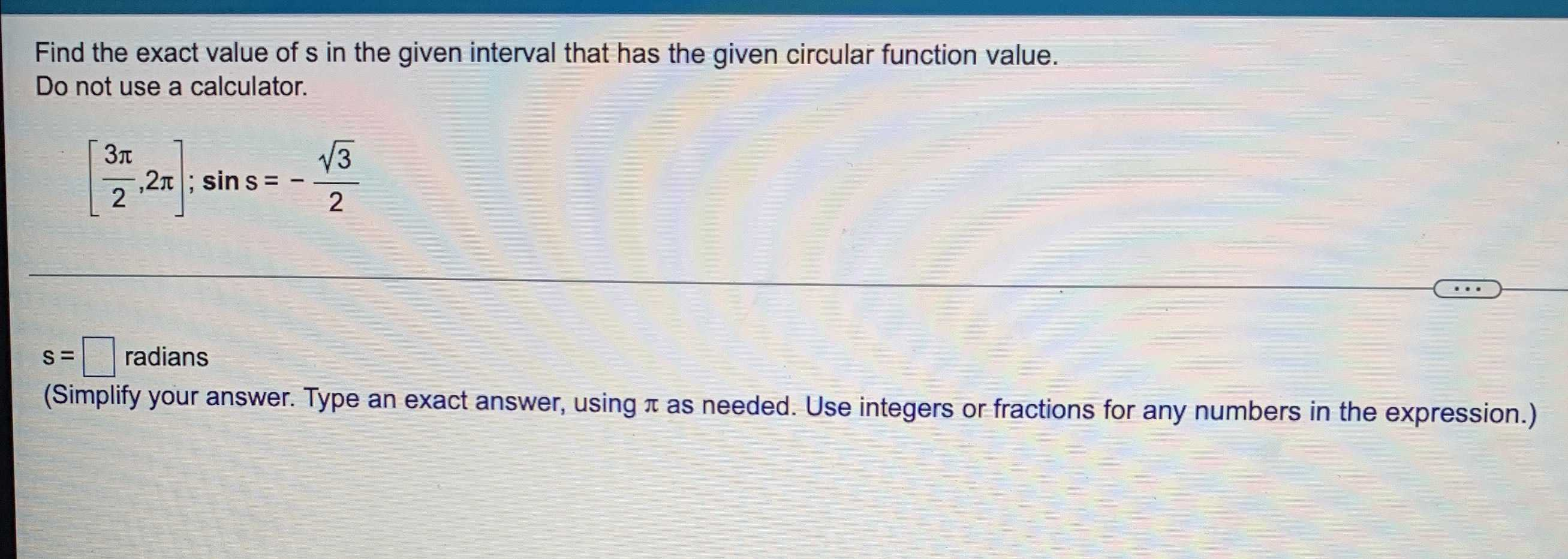### Still have math questions?

Trigonometry
QuestionFind the exact value of $$s$$ in the given interval that has the given circular function value. Do not use a calculator.

$$[ \frac { 3 \pi } { 2 } , 2 \pi ] ; \sin s = - \frac { \sqrt { 3 } } { 2 }$$

$$s = \square$$ radians (Simplify your answer. Type an exact answer, using $$\pi$$ as needed. Use integers or fractions for any numbers in the expression.)

$$\frac{5\pi }{3}$$